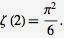## Thursday, 13 February 2014

### 1+2+3+4+...=-1/12

The equation

1+2+3+4+...=-1/12

is shorthand for how to meromorphically continue the Riemann zeta function beyond its region of convergence.

If you add up the integers there is no way you will get anything other than infinity. The equation needs to be understood in its context. The Riemann zeta function is defined asIf you put s equal to any number bigger than 1 then the infinite sum of fractions converges. For example put s=2 and the function says add together 1+1/4+1/9+1/16+... This infinite series can be added up and you get rather beautifullyHowever if you put s equal to a number less than 1 the infinite series doesn't make sense. For example put s=-1 and you get 1+2+3+4+...

But if you now allow s to be a complex number (not just a real number) then there is a unique way to smoothly extend the definition of the function into the region where it doesn't converge. It is essentially taking another formula which will make sense for values of s less than 1 but which seamlessly meshes with the function you have already defined. It is essential to put this in the context of complex numbers because we have a theorem which says there is a unique way to do this. So if you can extend the function there is only one way to do it. This new function gives the value -1/12 when you put in s=-1.

The story of this equation is discussed more in chapter 4 and chapter 6 of The Music of the Primes.

Do not trust physicists bearing divergent series. You will lose your wallet.